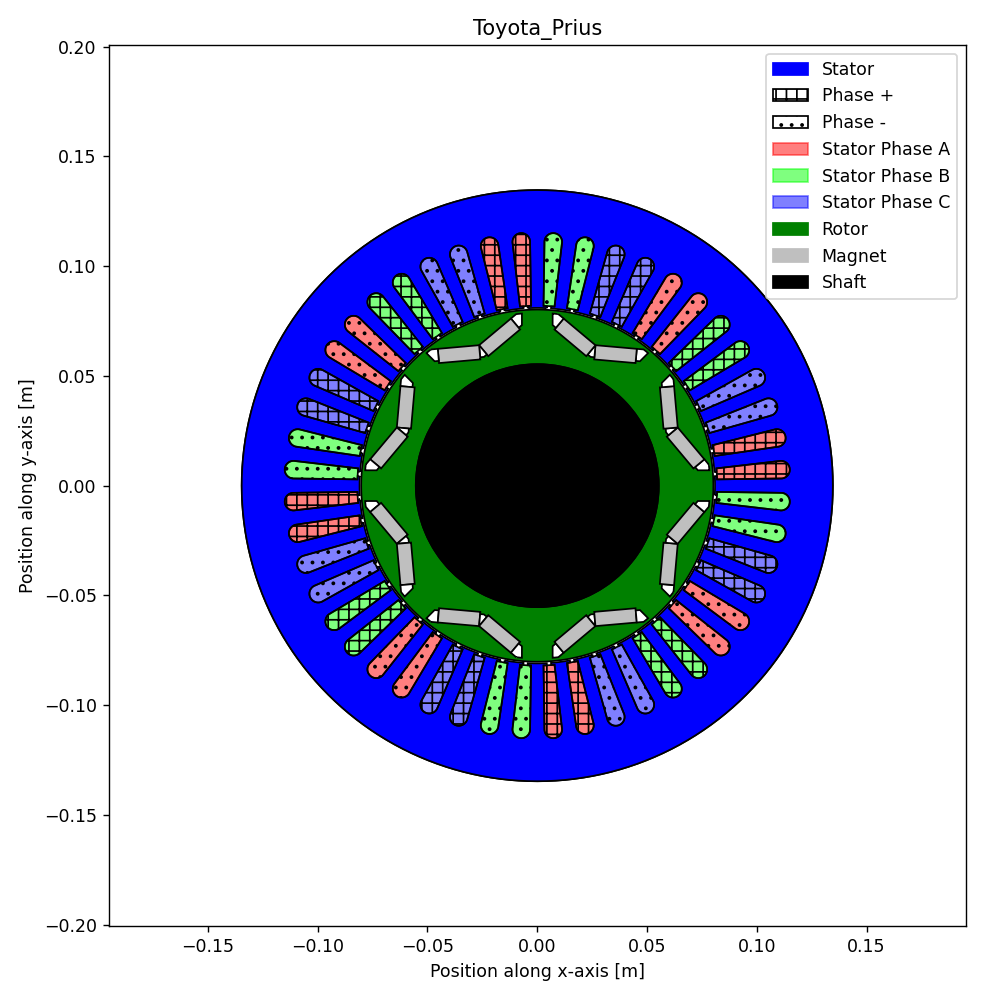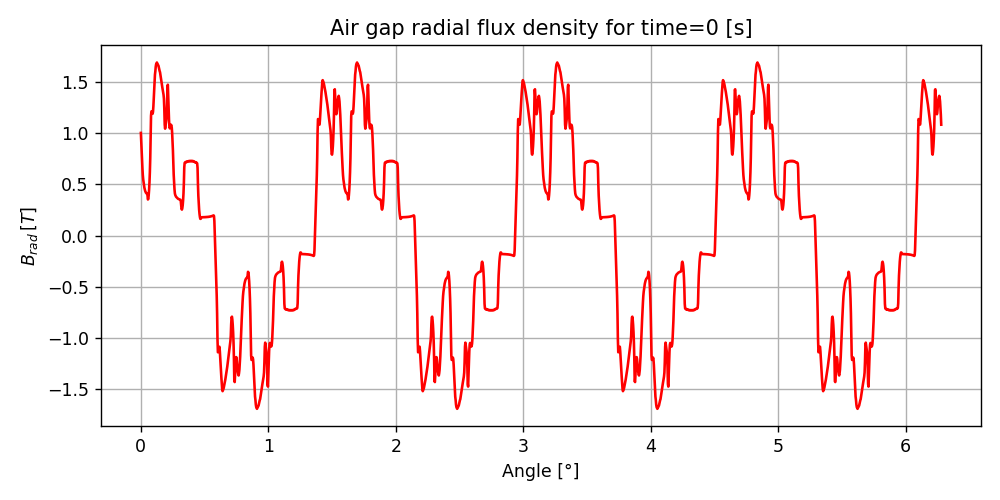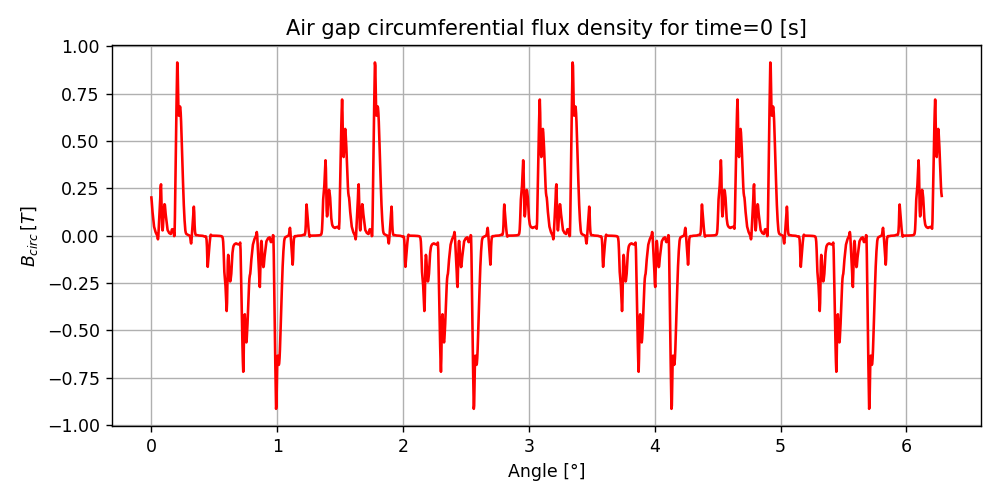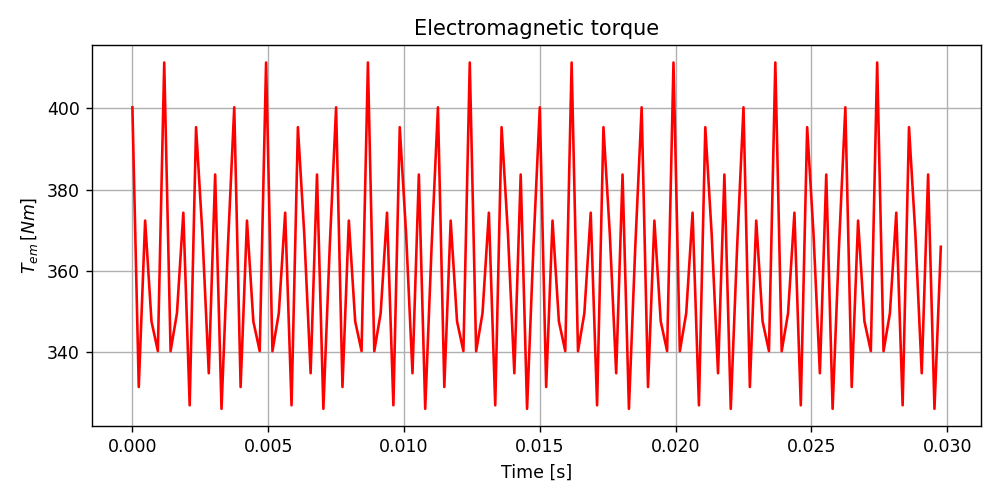# Version information¶

:

%matplotlib notebook
from PySide2.QtWidgets import *
from datetime import date
print("Running date:", date.today().strftime("%B %d, %Y"))
import pyleecan
print("Pyleecan version:" + pyleecan.__version__)
import SciDataTool
print("SciDataTool version:" + SciDataTool.__version__)

Running date: April 27, 2023
Pyleecan version:1.5.0
SciDataTool version:2.5.0


# How to set the Operating Point¶

This tutorial explains how to use the object OPdq, InputCurrent and VarLoadCurrent to run a magnetic simulation on several operating points by setting Id/Iq or I0/Phi0.

The reference used to validate this tutorial is: Z. Yang, M. Krishnamurthy and I. P. Brown, “Electromagnetic and vibrational characteristic of IPM over full torque-speed range,” 2013 International Electric Machines & Drives Conference, Chicago, IL, 2013, pp. 295-302, doi: 10.1109/IEMDC.2013.6556267.

## Machine and Simulation definition¶

This tutorial use the machine Toyota_Prius (2004) defined in the “How to define a machine” tutorial. The magnetic module is the same as the symmetrical one from the tutorial “How to define a simulation to call FEMM”.

:

%matplotlib notebook
from pyleecan.definitions import DATA_DIR
from os.path import join

fig, ax = Toyota_Prius.plot():

from pyleecan.Classes.Simu1 import Simu1
from pyleecan.Classes.MagFEMM import MagFEMM
# Initialization of the Simulation
simu_op = Simu1(name="tuto_Id_Iq", machine=Toyota_Prius)

# Definition of the magnetic simulation (FEMM with symmetry and sliding band)
simu_op.mag = MagFEMM(
type_BH_stator=0,
type_BH_rotor=0,
is_periodicity_a=True,
is_periodicity_t=True,
nb_worker=4,
Kgeo_fineness=1,
)
# Run only Magnetic module
simu_op.elec = None
simu_op.force = None
simu_op.struct = None


## Defining an Operating point with Id/Iq¶

The InputCurrent object enable to create an “OutElec” object that corresponds to the output of the Electrical module and the input of the Magnetic module. In this example, the Operating Point is set with an OPdq object to define the starting point with a sinusoidal current defined with Id_ref and Iq_ref:

The tutorial “How to define a simulation to call FEMM” uses the same InputCurrent object to enforce any current by directly setting Is

:

from pyleecan.Classes.InputCurrent import InputCurrent
from pyleecan.Classes.OPdq import OPdq
from numpy import sqrt, exp,pi

# Definition of a sinusoidal current
simu_op.input = InputCurrent()
# I0, Phi0 to set
I0_rms = 250/sqrt(2) # Maximum current [Arms]
Phi0 = 140*pi/180  # Maximum Torque Per Amp
# Compute corresponding Id/Iq
Id_ref = (I0_rms*exp(1j*Phi0)).real # [Arms]
Iq_ref = (I0_rms*exp(1j*Phi0)).imag # [Arms]
# Setting the values
simu_op.input.OP = OPdq(Id_ref=Id_ref, Iq_ref=Iq_ref)

(Id_ref,Iq_ref)

:

(-135.4188051049254, 113.62986941801093)


The discretization of the current and for the magnetic computation can be set with time and angle (as in “How to define a simulation to call FEMM” tutorial) or by setting the following parameters:

:

simu_op.input.Nt_tot = 128 # Number of time step
simu_op.input.Na_tot = 2048 # Spatial discretization
simu_op.input.OP.N0 = 2000 # Rotor speed [rpm]


When Nt_tot is defined, the time vector is automatically set to:

linspace(0, 60 / N0 * Nrev, Nt_tot)


With Nrev the number of revolution of the rotor (1 by default)

When Na_tot is defined, the angle vector is automatically set to:

linspace(0, 2*pi, Na_tot)


The input is now fully defined, the simulation can now be run:

:

from pyleecan.Functions.Plot import dict_2D

out_op = simu_op.run()

[15:57:36] Starting running simulation tuto_Id_Iq (machine=Toyota_Prius)
[15:57:36] Starting Magnetic module
[15:57:38] Computing Airgap Flux in FEMM
[15:57:45] End of simulation tuto_Id_Iq

:

# Plot the flux
out_op.mag.B.plot_2D_Data("angle", **dict_2D)
# Plot the torque
out_op.mag.Tem.plot_2D_Data("time", **dict_2D)
# Plot the current
out_op.elec.get_Is().plot_2D_Data("time", "phase[]", **dict_2D)